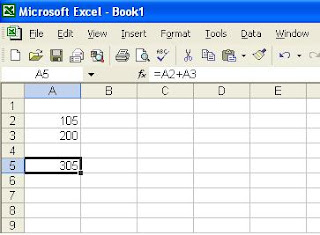## Friday, September 19, 2008

### Find sin(30) = 0.5

Do you have difficulty find the sine of degree / sin(degree) ?

I'll show you how ...

see picture belowFor example : you want to get sin(30)
Step 1 : You have to convert the number(30) to radian first.
Step 2 : Add formula "sin" in front of previous formula
Note : Don't forget to add bracket () for each formula
Tips : You can change the value of 30 to any cell, like A5
so if you change any value in cell A5,the result will change

then ... how if you want to find the opposite Asin(0.5)=30 ?

see picture below ...Step 1 : We use "ASIN" formula, type "=ASIN(0.5)"
Step 2 : Add "DEGREES" formula in front of previous formula
"=DEGREES(ASIN(0.5))" .... don't forget the bracket

Done ....!

## Thursday, September 18, 2008

### F2 Button trick

Do you know that you can edit your formula in excel by using F2 ???

I'll show you how ..

see the picture belowFor example you already insert 105 in cell A2 & 200 in cell A3.
Then you have typed formula " =A2+A3 " in cell A5.
If you want to edit the formula ......

Here's the step :
Step 1
: Put your cursor on the cell
Step 2 : Press F2 button ...... look the picture belowNow you can edit the formula ....... done !